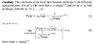# Local limit theorem

ehrenfest

## Homework Statement

This theorem is from Shiryaev. Can someone PLEASE explain how they are using the little o notation here. It makes no sense to me how they say that a FIXED real number is little o of something. I thought f(n) = o(g(n)) mean that for ever c>0 there exists an n_o such that when n => n_0, |f(n)|<c|g(n)|.

## The Attempt at a Solution

#### Attachments

•shiryaev_pg59.jpg
16.8 KB · Views: 376

Staff Emeritus
Gold Member
It makes no sense to me how they say that a FIXED real number is little o of something.
Where did they say that?

ehrenfest
Where did they say that?

It says for all $$x \in R^1$$

Staff Emeritus
Gold Member
It says for all $$x \in R^1$$
That doesn't sound like a FIXED real number to me. And besides, they clarify what they mean immediately afterwards.

Last edited:
ehrenfest
That doesn't sound like a FIXED real number to me.

They say for all $$x \in R^1$$ such that $$x = o(npq)^{1/6}$$. To me that means that you can take any real number that you want and see if it is $$o(npq)^{1/6}$$. I don't know how else to interpret it.

Staff Emeritus
Gold Member
I don't know how else to interpret it.
How about exactly how they interpreted it in the attachment you posted?

ehrenfest
How about exactly how they interpreted it in the attachment you posted?

That is precisely my question: How did they interpret it?

Staff Emeritus
Gold Member
How did they interpret it?
as $n \rightarrow \infty$,
$$\sup_{\left\{ x : |x| \leq \psi(n) \right\}} \left| \frac{P_n(np + x \sqrt{npq})}{ \frac{1}{\sqrt{2\pi npq}} e^{-x^2 / 2} } - 1 \right| \rightarrow 0,$$​
where $\psi(n) = o(npq)^{1/6}$.

ehrenfest
as $n \rightarrow \infty$,
$$\sup_{\left\{ x : |x| \leq \psi(n) \right\}} \left| \frac{P_n(np + x \sqrt{npq})}{ \frac{1}{\sqrt{2\pi npq}} e^{-x^2 / 2} } - 1 \right| \rightarrow 0,$$​
where $\psi(n) = o(npq)^{1/6}$.

OK. I will have to think about that. I am kind of confused about the sup above. Is \psi(n) fixed? If not, I don't understand how the sup is taken. Is it taken over all x AND over all \psi(n) that are $$o(npq)^{1/6}$$?

ehrenfest
anyone?

ehrenfest
I FINALLY figured this out! Hurky! was right that they clarify what they mean-that just wasn't clicking for me.